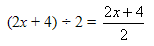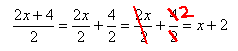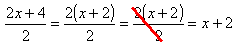Dividing Polynomials

Division of polynomials closely resembles simplifying fractions as demonstrated below:

ExampleThere are two ways to proceed. Split the division into two fractions, each with only one term on top, and then reduce:...or, find the Greatest Common Factor (GCF) and factor it from the numerator and denominator, and cancel common factor(s):Either way, the solution is the same: x + 2.